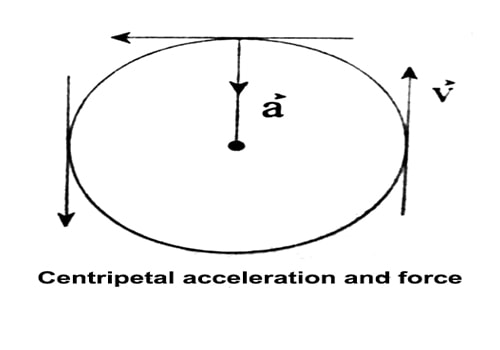# What is centripetal acceleration and centripetal force?

Centripetal acceleration:

When a point object is moving on a horizontal circular path with a constant speed, the direction of its velocity vector is changing with time. It means that uniform circular motion is an accelerated motion. When the object moves with uniform circular motion along the circumference of a circle, it is called uniform circular motion. In this case, there is no change of velocity of the object.

Acceleration acting on the object undergoing uniform circular motion is called centripetal acceleration. It always acts on the object along the radius towards the center of the circular path.Mathematically, it is expressed as,

a = v²/r = ω²r;

Where r is the radius of the circular path and ω and v are uniform angular and linear speed of the body.

Centripetal force:

The external force required to make a body moves along a circular path with uniform speed is Called Centripetal force.

When a point object (or rigid body) moves along a circular path with uniform speed, its direction changes continuously. Due to inertia, at every point of the circular path, the body tends to move along the tangent to the circular path at that point. According to Newton’s second law of motion, a change in the direction of motion of the body can take place only, if some external force acts on the body. Thus to move a body along a circular path, an external force is required, which is called centripetal force and is directed along the radius of the circular path and towards the center.

Mathematically, the centripetal force required to move a body of mass m along a circular path of radius r is given by,

F = mv²/r = mω²r